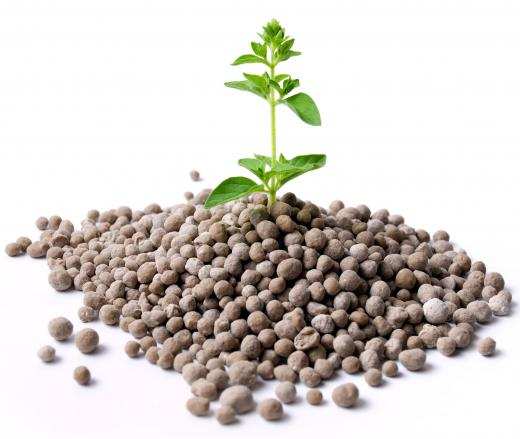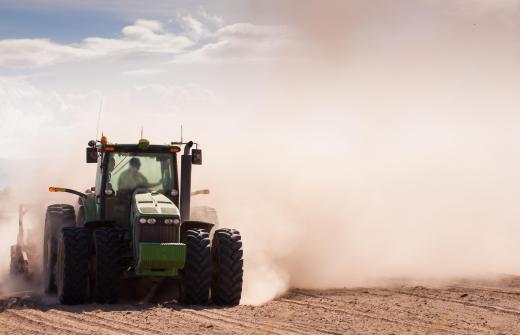Science
Fact Checked

# What Is a Variance?

Matt Hubbard
Matt Hubbard

Variance, like range, is a statistic related to the spread of a given sample or population. It is calculated for a given population by summing the squares of the difference between each element and the average, then dividing that total by the number of elements in the population. The more tightly a population is clustered around the mean, the lower the variance will be.

A closely related statistic is the standard deviation, which is the square root of the variance. The standard deviation is more frequently used in descriptive statistics because it is more intuitive and shares the same units as the mean. In the normal distribution, which is the classic bell-shaped distribution curve common to many phenomena, a little more than 95 percent of the population will lie within two standard deviations of the mean.A variance analysis might be used to determine the success of a newly formulated fertilizer compared to the existing variety.

Variance is most useful for predictive statistical techniques such as regression or analysis of variance (ANOVA). Regression will model a variable as the sum of one or more factors that influence the variable and the variance, which represents the difference between the actual observed elements and their expected values. For example, construction employment in a city might be modeled as a base level, plus a seasonal adjustment for time of year, plus an adjustment for the national economy, plus the variance. Regression techniques attempt to determine a model with the smallest variance, so that the expected value of the prediction hopefully will be close to observed value after observation is possible.An analysis of variance might be used in farming when testing a new fertilizer.

ANOVA, commonly used in clinical trials, is a statistical technique to classify sources of variance. Observations are categorized by one or more factors of interest in an experiment. Least squares techniques are used to partition the variance into random error, factor effects and interaction effects, with the goal of determining the influence that the factor or factors have on the variable. For example, a company that is testing a new fertilizer might use an ANOVA experiment with crop yield as the variable studied and factors of which fertilizer was used and how much rainfall the crops received. How the new fertilizer compared with other fertilizers would be a factor effect in the experiment; if the new fertilizer outperformed its rivals for standard rainfall but not for heavy rainfall, that would be an example of an interaction effect.

## You might also LikeFeryll

@Sporkasia - The use of the variance formula is pretty common in fields that measure population diversity. Variance can be a useful tool for politicians seeking election as they try to predict the issues that will be important to the people who will be voting for them or their opponents.

Sporkasia

The concept of variance can be a bit difficult to understand. When we studied this in my high school math classes I thought the variance formula was a bit of useless information created for the sole purpose of tormenting math students. I thought I would never see the formula once I left school and entered the real world. Now I am brushing up on the formula for a work project. I really didn't see this coming.